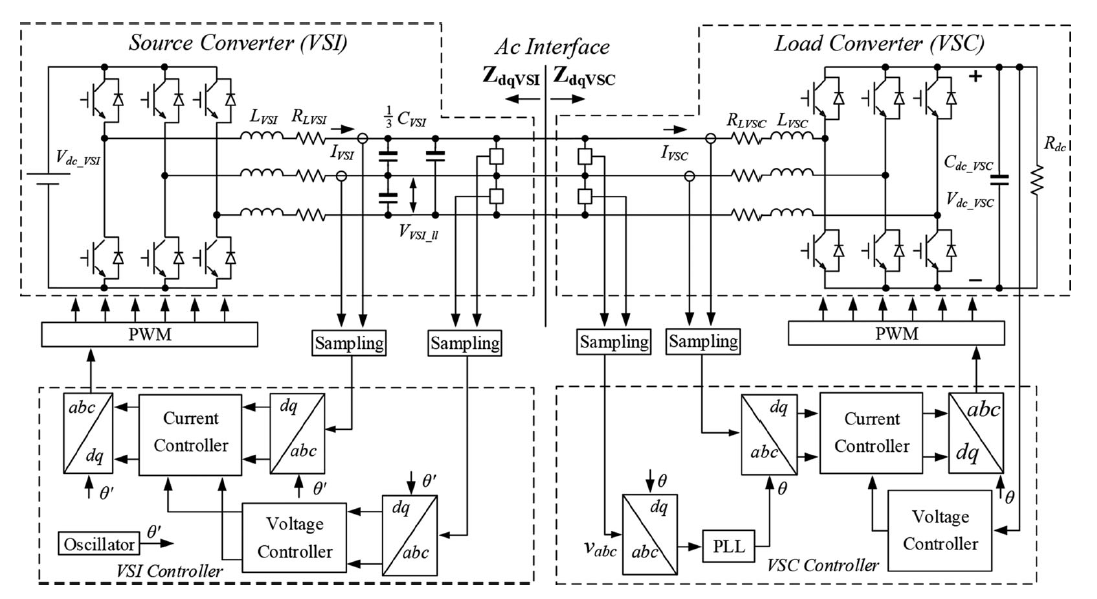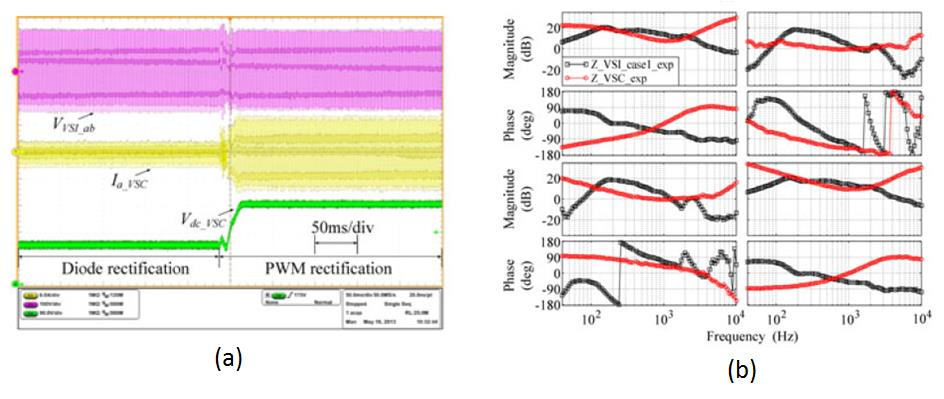LIBRARY

# Small-Signal Stability Analysis of Three-Phase AC Systems in the Presence of Constant Power Loads Based on Measured d-q Frame ImpedancesFig. 1. Experimental system setup and control scheme
Small-signal stability is of great concern for electrical power systems with a large number of regulated power converters. In the case of dc systems, stability can be predicted by examining the locus described by the ratio of the source and load impedances in the complex plane per the Nyquist stability criterion. In the case of three-phase ac systems, the impedance-based stability can be formulated using the synchronous d-q frame source and load impedances. Recently, the use of the generalized Nyquist stability criterion (GNC) was proposed to predict the stability at the ac interfaces in the d-q frame. This paper studies the small-signal stability of an ac system comprised of a voltage-source inverter (VSI) feeding a boost rectifier or voltage-source converter (VSC), in what is regarded to be a very generic system configuration.

The paper discusses how stable and unstable cases are created in the experimental system, shown in Fig. 1, based on the derived d-q frame average model of both the VSI and VSC. The constant power loads and instability conditions are also considered, but solely through the d-d channel. The d-q frame impedance measurement techniques and algorithm are then discussed. The experimental results, including time-domain waveforms and measured d-q frame impedances, like Fig. 2, validate all stability conditions. Because of its accuracy, using this approach to predict stability in both the frequency domain and time domain has the potential to enable the use of impedance specification as a design tool for future ac systems.Fig. 2. Measurement results of one case: (a) Time-domain results; (b) d-q impedance